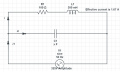# RLC AC circuit analysis find value of Capacitor

#### Juan Nicolas Pardo Martin

Joined May 28, 2018
1This is a sketch I did of the excercise, basically I know a few things about the Circuit.
The excercise says that the power factor should be 0.9, given that find the Capacitance, We know that the phase shift of the RL part of the circuit is around 43,3°
We know the effective current i, but we know i1=ic+i, right?
i=1.67a (the current only on the resistance-inductor part)
(I do know that I'm supposed to get Capacitance as 7.7µF) But I have not yet understood very well how to get there.
Please let me know if this thought process is correct:
w = 50 * 2* Pi (angular velocity)
1 This is a parallel circuit between two Impedances, we know one, and we don't know the other.
First impedance is
Z_1=100+j(30*Pi)
Second Impedance is:
Z_2=-j(1/100*Pi*C)
Total Impedance would be
Z_3=1/(1/Z_1+1/Z_2) right?
Then the cosine of the angle in polar coordinates would be 0.9
However I've replaced this result C=7.7µF on Mathematica and I get a wildly different result, leading me to think this is not how you solve this problem

Well I have Z_1 and I have voltage, but I only have their amplitudes.. am I missing some data?
What should I do, what should I research?

#### MrAl

Joined Jun 17, 2014
7,465
View attachment 153255
This is a sketch I did of the excercise, basically I know a few things about the Circuit.
The excercise says that the power factor should be 0.9, given that find the Capacitance, We know that the phase shift of the RL part of the circuit is around 43,3°
We know the effective current i, but we know i1=ic+i, right?
i=1.67a (the current only on the resistance-inductor part)
(I do know that I'm supposed to get Capacitance as 7.7µF) But I have not yet understood very well how to get there.
Please let me know if this thought process is correct:
w = 50 * 2* Pi (angular velocity)
1 This is a parallel circuit between two Impedances, we know one, and we don't know the other.
First impedance is
Z_1=100+j(30*Pi)
Second Impedance is:
Z_2=-j(1/100*Pi*C)
Total Impedance would be
Z_3=1/(1/Z_1+1/Z_2) right?
Then the cosine of the angle in polar coordinates would be 0.9
However I've replaced this result C=7.7µF on Mathematica and I get a wildly different result, leading me to think this is not how you solve this problem

Well I have Z_1 and I have voltage, but I only have their amplitudes.. am I missing some data?
What should I do, what should I research?

Hello,

Are you doing the calculation correctly? I get 0.8995 which is close enough to 0.9 for me.